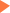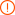# 100个SAT数学真题中的高频词汇合集

2014年11月26日16:32 来源：小站整理## SAT/ACT备考资料免费领取 请选择资料！

SAT数学部分的考试对于中国考生来说可能难度不大，也因此有很多学生会忽略数学考试，这样是很不对的。下面就来给大家介绍一下SAT数学真题中总结的一些高频词汇，希望大家有所了解。

abscissa 横坐标

absolute value 绝对值

account for （数量）占

acute angle 锐角

acute triangle 锐角三角形

algebra 代数学

algebraic expression 代数式

algebraic fraction 分式

aliquant 除不尽数

alternate angles 内错角

altitude 高度

apiece 每人，每个

approximately 近似的，大约的

approximation 近似，近似值

arc 弧，圆周的任意一段

area 面积

arithmetic 算术

arithmetic（al） average 算术平均数

arithmetic（al） mean 算术平均数或等差中项

at random 随机地

at right angles with 与……成直角

be composed of 由构成

be equal to 与……相等

be equivalent to anther equation 与另一方程痛解

be fewer than 小于

be greater than 大于

be greater than or equal to 不小于

be inscribed in 内接于

be less than 小于

be perpendicular to 垂直于

calculate to three decimal places 计算结果保留三位小数

cancellation 约掉，消掉

common difference 等差数列的公差 留学

common ratio 等比数列的公比

common multiple 公倍数

complement 余角

complementary angle 余角

complete quadratic （equation） 全二次方程

complex fraction 繁分数

composite number 和数，指大于一而不是质数的整数

compound 混合物；

decimal place 小数位

decimal point 小数点

decrease 减少

degree 度；度数（温度和角度）

denominator 分母

equality 相等，等式

equation 等式，方程

equiangular 等角的

equidistance 等距离的

even integer 偶数

### 专题推荐未输入验证码未输入验证码未输入验证码

## SAT30天热搜沪公网安备 31010602002658号